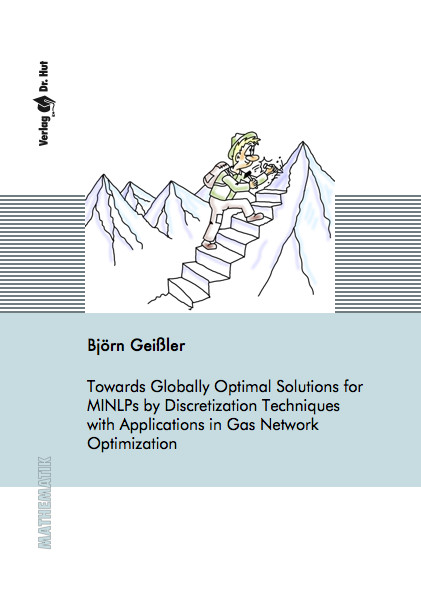Datenbestand vom 20. August 2019aktualisiert am 20. August 2019

# ISBN 9783843901680Euro 84,00 inkl. 7% MwSt

978-3-8439-0168-0, Reihe Mathematik

Björn Geißler
Towards Globally Optimal Solutions for MINLPs by Discretization Techniques with Applications in Gas Network Optimization

155 Seiten, Dissertation Universität Erlangen-Nürnberg (2011), Hardcover, A5

## Zusammenfassung / Abstract

In this thesis we develop techniques which turn any general purpose MIP-solver into a general purpose solver for MINLP-problems. To this end we carry over the idea of solving LP-relaxations from mixed-integer linear programming to the case of mixed-integer nonlinear programming. In order to solve MINLP-problems we show how to construct arbitrary tight MIP-relaxations of the underlying nonlinear problem. For the construction of the MIP-relaxations we extend well-known MIP-techniques for piecewise linear functions and make use of convex underestimators to construct these relaxations such that any point which is feasible for the relaxation satisfies all nonlinear constraint up to some arbitrary small, a priori given error bound. In addition we present an algorithm which iteratively solves adaptively refined MIP-relaxations in order to determine the global optimum of a given MINLP.

Thereafter we derive an MINLP-model for the gas network nomination validation problem, where the aim is to decide whether the controlable elements of a given gas network can be operated such that the demands of all customers are satisfied. Complementing this MINLP-model by a suitable objective function yields the gas network energy cost minimization problem. Computational experiments are carried out for small, medium-sized and even large-scale real-world instances of both problems. Our numerical results show that MIP-relaxations are particularly useful in solving these problems. Moreover, we will see that by applying the techniques developed in this work, it is possible to solve either of these problems by using an MIP-solver only.